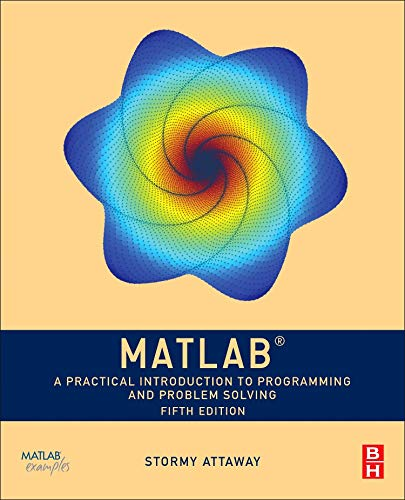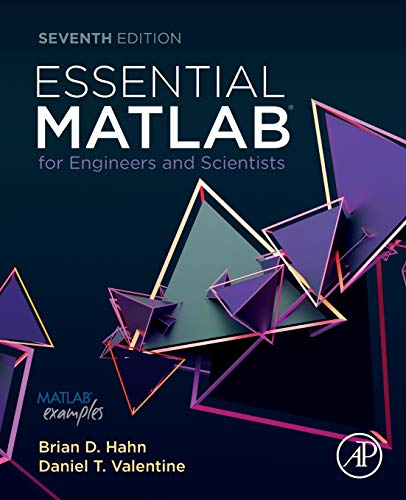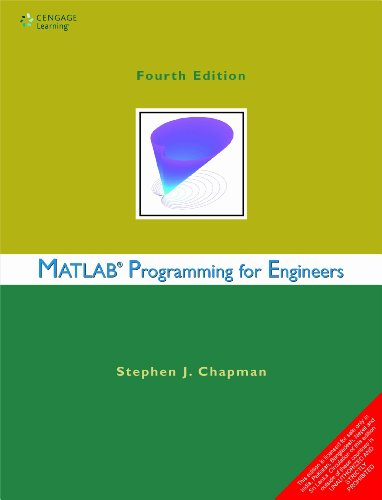# Best MatLab Books to Develop Algorithms and Solve Computing Problems

MatLab is a matrix-based programming language and is a platform that is specifically designed for engineers and scientists. MatLab can be used to solve many technical computing problems including modeling, simulation, prototyping, data analysis, exploration, visualization, scientific and engineering graphics.

Here you will get some of the best Matlab books.

## 1. MATLAB: A Practical Introduction to Programming and Problem SolvingAuthor: Stormy Attaway Ph.D. Boston University
Published at: 17/08/2018
ISBN: 0128154799

A Practical Introduction to Programming and Problem Solving is very easy to understand and shows you an excellent way to learn Matlab on your own. It's very good coverage of the basics, more advanced topics with plenty of trial examples at the end of each chapter and is a great book that presents programming concepts and MATLAB built-in functions side-by-side.

What you will learn

• Programming concepts such as variables, assignments, input/output, and selection statements

• An input/output topic which is fundamental to many engineering applications

• Vectorized code to emphasize the importance of using MATLAB efficiently

• Low-level file input functions

• Graphical user interfaces

• Improved standards for variable names and documentation.

## 2. MATLAB:INTRO.WITH APPLICATIONS

This is a great introductory and very informative handbook for anyone who wants to know how to work with vectors and matrices, write simple programs and function files, plot and format data, fit data to a curve, write commands and scripts and how to differentiate and integrate both numerically and symbolically.

This book covers the following topics

• Creating array

• Mathematical operations with arrays

• Using script files and managing data

• Two-dimensional plots

• Programming in MATLAB

• User-defined functions and function files

• Polynomials, curve fitting, and interpolation

• Applications in numerical analysis

• Three-dimensional plots

• Symbolic math

• Characters, commands, and functions

## 3. MATLAB for Engineers (5th Edition)

MATLAB for Engineers is an excellent reference book with lots of engineering examples and can be used to solve a wide range of engineering problems. It provides a vast world of matrix manipulation software that will guide you in solving practical problems with Matlab with a step-by-step approach that helps to clarify your problem-solving.

Key topics include

• Built-In MATLAB functions

• Manipulating MATLAB matrices

• Plotting

• User-defined functions

• User-controlled input and output

• Logical functions and selection structures

• Repetition structures

• Matrix algebra and other kinds of arrays

• Symbolic mathematics

• Numerical techniques

• Creating graphical user interfaces

• A brief introduction to Simulink.

## 4. MATLAB For Dummies

MATLAB  for Dummies is an excellent and well-written book for both the experienced and the novice to automate their work with programming scripts and functions. This book gives you the information about how to use MatLab, plot graphs in 2D and 3D to visualize your data, linear algebra, language fundamentals including syntax, operators, and data types.

This book consists of the following six parts

• Getting started with MATLAB

• Manipulating and plotting data in MATLAB

• Streamlining MATLAB

• Specific MATLAB applications

• The part of tens

## 5. Accelerating MATLAB Performance: 1001 tips to speed up MATLAB programs

A very good book with good examples supported by an active website contains GPU, MEX, FPGA, and other forms of compiled code, techniques for speeding up deployed applications universe of real-life, MATLAB vectorization, parallelization (implicit and explicit), optimization, memory management, chunking, and caching and improve your coding skills for taking you to the next level.

You'll learn the following things

• The way of attaining significant reductions in development costs and program run times.

• Specific tips for MATLAB GUI, graphics, and I/O

• A wide variety of utilities, libraries, and toolboxes that can help to improve performance

• You can apply a huge amount of performance-related solutions in real-life projects

• Also contains a lot of code snippets compiled by the author to help readers for implementing their own projects

• An appendix that covers MATLAB usage and pertinent software engineering prospects/solutions

## 6. MATLAB for Engineering Applications

Introduction to MATLAB for Engineers is a good introductory textbook that translates MATLAB to help modern engineers and scientists. This book is a very useful resource for beginners who have little experience in programming.

Key topics include

• Numeric, cell, and structure arrays

• Functions and files

• Decision-making programs

• Model building and regression

• Linear algebraic equations

• Probability, statistics, and interpolation

• Numerical methods for calculus and differential equations

## 7. Essential MATLAB for Engineers and ScientistsAuthor: Brian Hahn,Daniel Valentine Ph.D.
Published at: 12/04/2019
ISBN: 0081029977

Essential MATLAB for Engineers and Scientists is a great reference book to learn the basics of Matlab with examples. This book presents the new H2 graphics features and a comparison of execution time between compiled and un-compiled code that includes examples with coverage of both the fundamentals and applications.

Coverage Includes

• MATLAB fundamentals

• Program design and algorithm development

• MATLAB functions and data import-export utilities

• Logical vectors

• Matrices and arrays

• MATLAB graphics

• Vectors as arrays and other data structures

• Errors and pitfalls

• Applications and simulation

• Introduction to numerical methods

• Signal processing

• Symbolics toolbox

## 8. Digital Image Processing using MATLAB: ZERO to HERO Practical Approach with Source Code (Handbook of Digital Image Processing using MATLAB)

This is an excellent step by step approach to digital image processing with MATLAB. Content includes basic morphological operation, image segmentation, histogram equalization, spatial intensity resolution, image enhancement in spatial filtering, image enhancement in frequency filtering, color image processing, DFT analysis, DFT analysis, image sampling, and quantization and various image transformation with MATLAB source code.

What you will learn

• The importance of digital image processing and the necessity of image processing stems from application areas.

• The processing of a spectacle data for a machine perception

• Processing of x-rays, ultrasonic scanning, electron micrographs, magnetic resonance imaging, nuclear magnetic resonance imaging

• The image processing procedures like restoration and image enhancement

• How to solve the issue of a machine to attain superior results.

## 9. Matlab Programming For Engineers,4EdAuthor: STEPHEN J. CHAPMAN
Published at: 01/01/2012
ISBN: 8131518655

Stephen J. Chapman covers the basics of Matlab and several interesting examples in each chapter to highlight the details that allow you to quickly pick up MATLAB. He introduces the Matlab language and shows how to write clean, efficient, and well-documented programs and use it to solve typical technical problems. This book is easy to read and the information is presented in a logical sequence and fully explained and illustrated.

You'll learn

• Programming and Matlab simultaneously
• Top-down design
• How to approach debugging programs including the use of the integrated debugger.

Contents include

• MatLab basics
• Branching statements and program design
• Loops
• User-defined functions
• Additional data types and plot types

Thanks for reading this post. If you have any opinion don't hesitate to comment here. Also please subscribe our newsletter to get more updates.